# Multiclass Object Detection Using YOLO v2 Deep Learning

This example shows how to perform multiclass object detection on a custom dataset.

### Overview

Deep learning is a powerful machine learning technique that you can use to train robust multiclass object detectors such as YOLO v2, YOLO v4, SSD, and Faster R-CNN. This example trains a YOLO v2 multiclass object detector using the `trainYOLOv2ObjectDetector` function. The trained object detector is able to detect and identify multiple indoor objects. For more information regarding training other multiclass object detectors such as YOLO v4, SSD, or Faster R-CNN, see, Getting Started with Object Detection Using Deep Learning.

This example first shows you how to detect multiple objects within an image using a pretrained YOLO v2 object detector. Then, you can optionally download a dataset and train YOLO v2 on a custom dataset using transfer learning.

```pretrainedURL = "https://www.mathworks.com/supportfiles/vision/data/yolov2IndoorObjectDetector23b.zip"; pretrainedFolder = fullfile(tempdir,"pretrainedNetwork"); pretrainedNetworkZip = fullfile(pretrainedFolder, "yolov2IndoorObjectDetector23b.zip"); if ~exist(pretrainedNetworkZip,"file") mkdir(pretrainedFolder); disp("Downloading pretrained network (6 MB)..."); websave(pretrainedNetworkZip, pretrainedURL); end unzip(pretrainedNetworkZip, pretrainedFolder) pretrainedNetwork = fullfile(pretrainedFolder, "yolov2IndoorObjectDetector.mat"); pretrained = load(pretrainedNetwork); detector = pretrained.detector;```

### Detect Multiple Indoor Objects

Read a test image that contains objects of the target classes, run the object detector, and display an image annotated with the detection results.

```I = imread('indoorTest.jpg'); [bbox,score,label] = detect(detector, I); annotatedImage = insertObjectAnnotation(I,"rectangle",bbox,label,LineWidth=4,FontSize=24); figure imshow(annotatedImage)```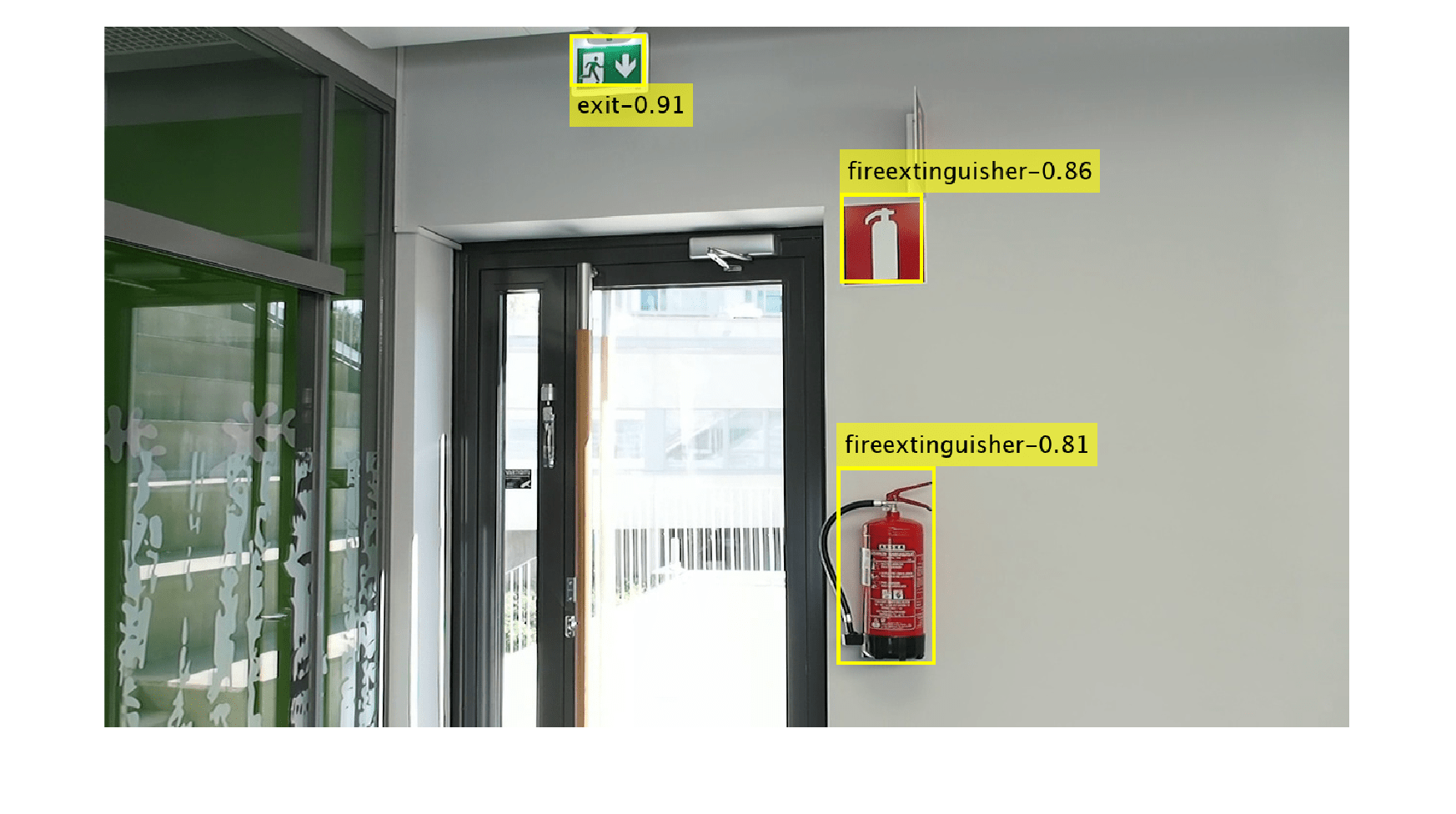This example uses the Indoor Object Detection dataset created by Bishwo Adhikari . The dataset consists of 2213 labeled images collected from indoor scenes containing 7 classes - fire extinguisher, chair, clock, trashbin, screen, and printer. Each image contains one or more labeled instances of these classes. Check whether the dataset is already downloaded and if it is not, then use `websave` to download it.

```dsURL = "https://zenodo.org/record/2654485/files/Indoor%20Object%20Detection%20Dataset.zip?download=1"; outputFolder = fullfile(tempdir,"indoorObjectDetection"); imagesZip = fullfile(outputFolder,"indoor.zip"); if ~exist(imagesZip,"file") mkdir(outputFolder) disp("Downloading 401 MB Indoor Objects dataset images..."); websave(imagesZip, dsURL); unzip(imagesZip, fullfile(outputFolder)); end```

Create an `imageDatastore` to load the data.

```datapath = fullfile(outputFolder, "Indoor Object Detection Dataset"); imds = imageDatastore(datapath, IncludeSubfolders=true, FileExtensions=".jpg");```

Annotations and dataset split have been provided in `annotationsIndoor.mat`. Load the annotations and the indices corresponding to the training, validation, and test sets. Note that the split contains 2207 images in total instead of 2213 images as 6 images have no labels associated with them. Store the indices of images containing labels in `cleanIdx`.

```data = load("annotationsIndoor.mat"); bbStore = data.BBstore; trainingIdx = data.trainingIdx; validationIdx = data.validationIdx; testIdx = data.testIdx; cleanIdx = data.idxs; % Remove the 6 images with no labels. imds = subset(imds,cleanIdx); bbStore = subset(bbStore,cleanIdx);```

### Analyze Training Data

Analyze the distribution of object class labels and sizes to understand the data better. This analysis is critical because it helps determine how to prepare the training data and how to configure an object detector for this specific dataset.

#### Analyze Class Distribution

Measure distribution of bounding box class labels in the dataset with `countEachLabel`.

`tbl = countEachLabel(bbStore)`
```tbl=7×3 table Label Count ImageCount ________________ _____ __________ exit 545 504 fireextinguisher 1684 818 chair 1662 850 clock 280 277 trashbin 228 170 screen 115 94 printer 81 81 ```

Visualize the counts by class.

```bar(tbl.Label,tbl.Count) ylabel("Frequency")```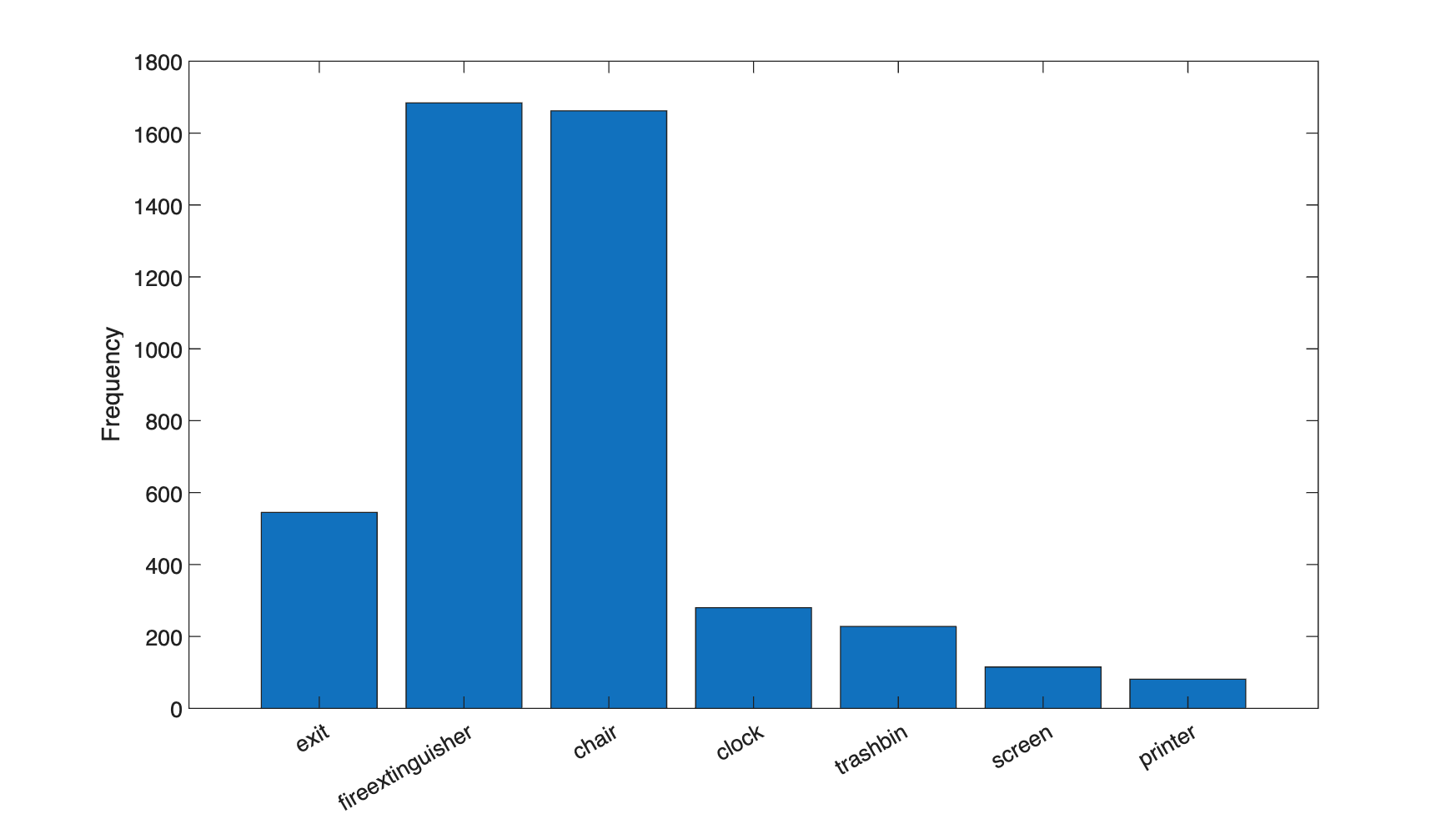The classes in this dataset are unbalanced. If not handled correctly, this imbalance can be detrimental to the learning process because the learning is biased in favor of the dominant classes. There are multiple, complementary, techniques used to deal will this issue - adding more data, oversampling the underrepresented classes, modifying loss function, and data augmentation. Each of these approaches require empirical analysis to determine the optimal solution. You will apply data augmentation in a later section.

#### Analyze Object Sizes

Read all the bounding boxes and labels within the dataset and calculate the diagonal length of the bounding box.

```data = readall(bbStore); bboxes = vertcat(data{:,1}); labels = vertcat(data{:,2}); diagonalLength = hypot(bboxes(:,3),bboxes(:,4));```

Group object sizes by class.

```G = findgroups(labels); groupedDiagonalLength = splitapply(@(x){x},diagonalLength,G);```

Visualize the distribution of object lengths for each class.

```figure classes = tbl.Label; numClasses = numel(classes); for i = 1:numClasses len = groupedDiagonalLength{i}; x = repelem(i,numel(len),1); plot(x,len,"o"); hold on end hold off ylabel("Object extent (pixels)") xticks(1:numClasses) xticklabels(classes)```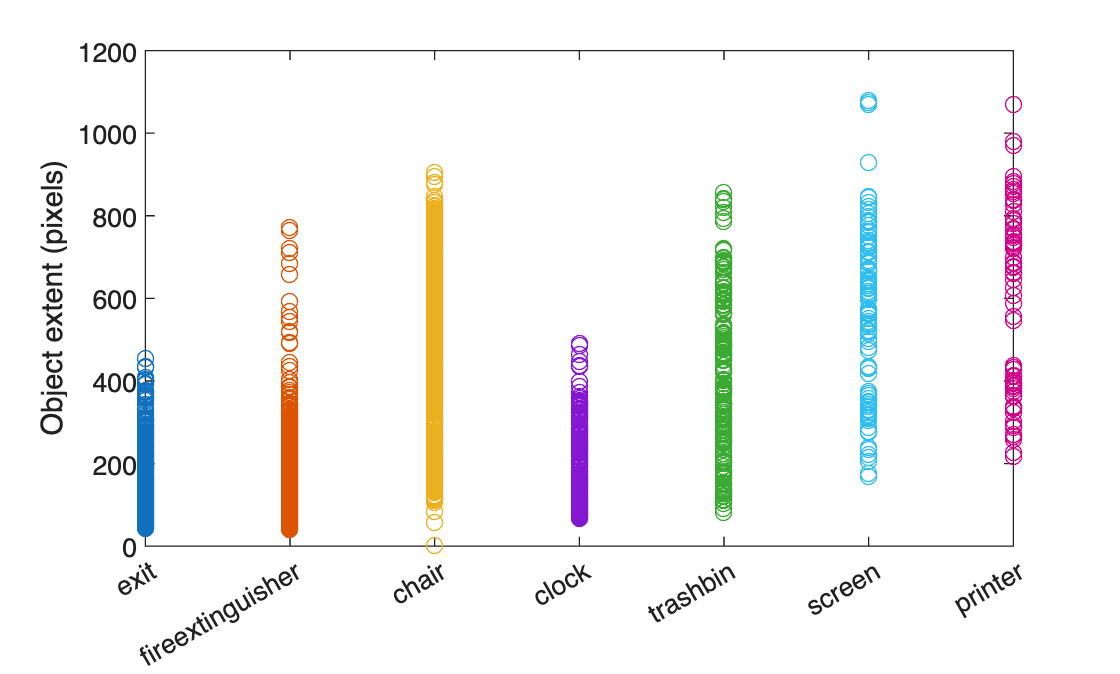This visualization highlights several important dataset attributes that help you understand the type of object detector to configure:

1. The object size variance within each class.

2. The object size variance across classes.

In this dataset, the there is a good amount of overlap between the size ranges across classes. In addition, the size variation within each class is not very large. This means that one multi-class detector can be trained to handle the range of object sizes. If the size ranges do not overlap or if the range of object sizes is more than 10x apart, then training multiple detectors for different size ranges is more practical.

The size variance also informs which object detector to train. Object detectors such as YOLO v2 are more likely to succeed when there is limited size variance within each class. If there is large variance within each class then choosing a multi-scale object detector such as YOLO v4 or SSD is a better choice. Given that the object sizes within this dataset are all within the same order of magnitude, YOLO v2 is a reasonable starting point. Although more advanced, multi-scale, detectors may perform better, it may take more resources and time to train compared with YOLO v2. Consider using more advanced detectors if simpler solutions do not reach your desired performance requirements.

In addition, the size distribution information helps select the training image size. Object detectors are typically trained at a fixed image size to enable batch processing during training. The training image size dictates how large the batch size can be during training given the resource contraints of your training environment (for example, GPU memory). Processing larger batches of data can improve throughput and reduce training time, especially on a GPU. However, the training image size may also impact the visibility of objects within those images if the original data is drastically resized to a smaller size.

You will use this size analysis information in the next section to configure YOLO v2 for this dataset.

### Configure a YOLO v2 Detector

Configure a YOLO v2 object detector using the following steps:

1. Chooose a pretrained detector for transfer learning.

2. Choose a training image size.

3. Select which network features to use for predicting object locations and classes.

4. Estimate anchor boxes from the preprocessed data used to train the object detector.

Select a pretrained Tiny YOLO v2 detector for transfer learning. Tiny YOLO v2 is a lightweight network trained on COCO , a large object detection dataset. Transfer learning from a pretrained object detector reduces the time it takes to train compared to training a network from scratch. The other pretrained detector is the larger Darknet-19 YOLO v2 pretrained detector. Consider starting with simpler networks to establish a performance baseline before experimenting with larger networks. Using Tiny or Darknet-19 YOLO v2 pretrained detectors requires the Computer Vision Toolbox Model for YOLO v2 Object Detection.

`pretrainedDetector = yolov2ObjectDetector("tiny-yolov2-coco");`

Next, choose the size of the training images for YOLO v2. When choosing the training image size, consider

1. The distribution of object sizes and the impact resizing the image will have on the object sizes.

2. The computational resources required to batch process data at the selected size.

3. The minimum input size required by the network.

Determine the input size of the pretrained Tiny YOLO v2 network.

`pretrainedDetector.Network.Layers(1).InputSize`

The size of the images within the Indoor Object Detection dataset is [720 1024 3]. Based on the object analysis done in the previous section, the smallest objects are approximately 20x20.

To maintain a balance between accuracy and computational cost of running the example, specify a size of [720 720 3]. This size ensures that resizing the image down will not drastically effect the spatial resolution of objects in this dataset. If you adapt this example for your own dataset, you must change the training image size based on your data. Determining the optimal input size requires empirical analysis.

`inputSize = [720 720 3];`

Use `transform` to apply a preprocessing function that will resize images and the bounding boxes. In addition, it also sanitizes the bounding boxes to convert them to a valid shape.

`preprocessedData = transform(ds,@(data)resizeImageAndLabel(data, inputSize));`

Display one of the preprocessed images and box labels to verify that the objects in the resized images still have visible features.

```data = preview(preprocessedData); I = data{1}; bbox = data{2}; label = data{3}; imshow(I) showShape("rectangle", bbox, Label=label)```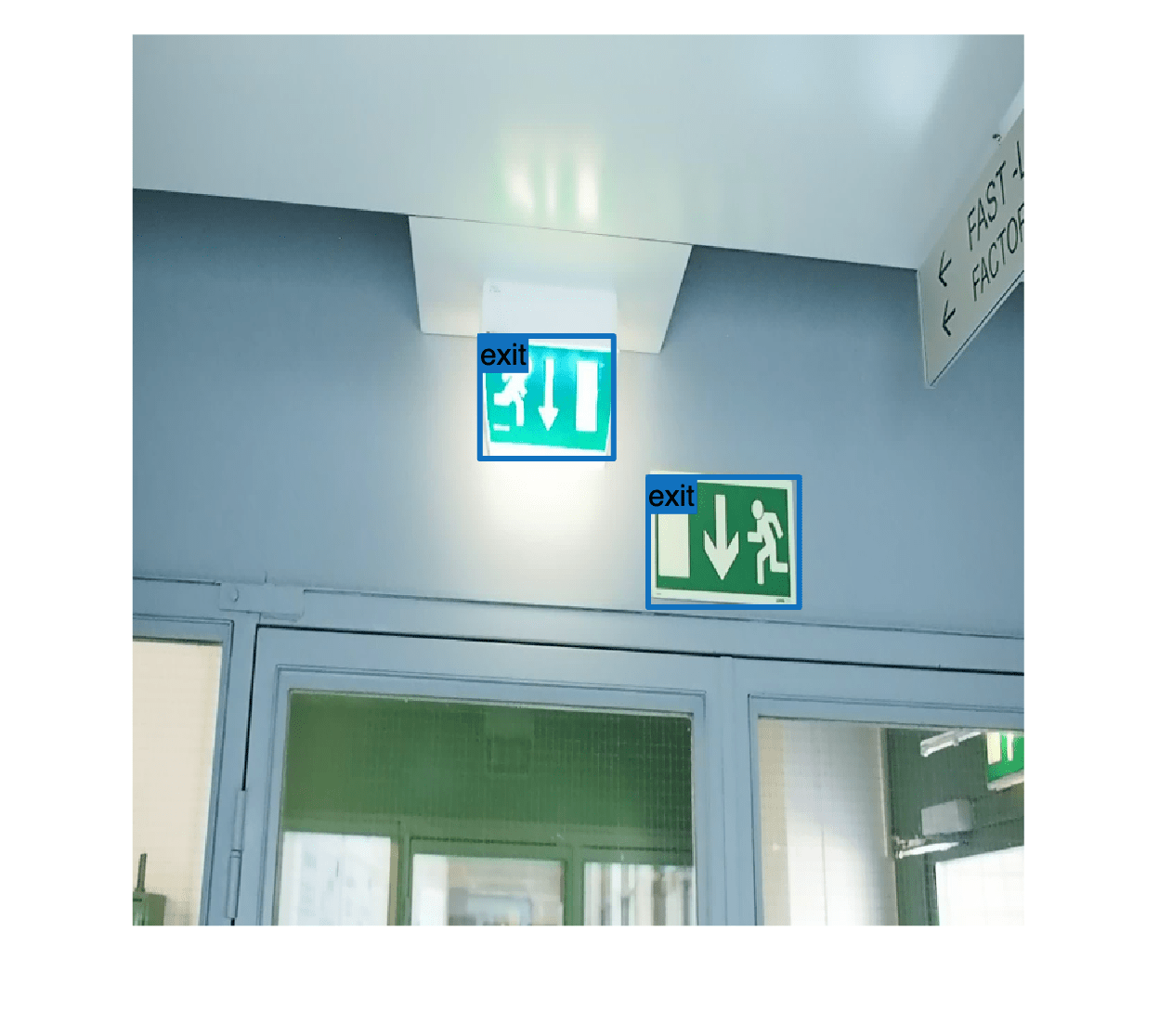YOLO v2 is a single-scale detector because it uses features extracted from one network layer to predict the location and class of objects in the image. The feature extraction layer is an important hyperparameter for deep learning based object detectors. When selecting the feature extraction layer, choose a layer that outputs features at a spatial resolution that is suitable for the range of object sizes in the dataset.

Most networks used in object detection spatially downsample features by powers of two as the data flows through the network. For example, starting at a given input size, networks will have layers that produce feature maps that are downsampled spatially by 4x, 8x, 16x, and 32x. If object sizes in the dataset are small, for example, less than 10x10, feature maps dowsampled by 16x and 32x may not have have sufficient spatial resolution to locate the objects precisely. Conversely, if the objects are large, feature maps downsampled by 4x or 8x may not encode enough global context for larger objects.

For this dataset, the layer named `"layer_relu_5"` is selected because the output feature maps are downsampled by 16x. This amount of downsampling is a good trade-off between spatial resolution and the strength of the extracted features, as features extracted further down the network encode stronger image features at the cost of spatial resolution.

`featureLayer = "leaky_relu_5";`

Note, `analyzeNetwork` was used to visuzliae the tiny YOLO v2 network and determine the name of the layer that outputs features downsampled by 16x.

Next, use `estimateAnchorBoxes` to estimate anchor boxes from the training data. You must estimate anchor boxes from the preprocessed data to get an estimate based on the selected training image size. Use the procedure defined in Estimate Anchor Boxes From Training Data to determine the number of anchor boxes suitable for this dataset. Based on this procedure, using 5 anchor boxes is a good trade-off between computational cost and accuracy. As with any other hyperparameter, the number of anchor boxes should be optimized using empirical analysis.

```numAnchors = 5; aboxes = estimateAnchorBoxes(preprocessedData, numAnchors);```

Finally, configure YOLO v2 for transfer learning on 7 classes with the selected training image size, and estimated anchor boxes.

```numClasses = 7; pretrainedNet = pretrainedDetector.Network; lgraph = yolov2Layers(inputSize, numClasses, aboxes, pretrainedNet, featureLayer);```

You can visualize the network using `analyzeNetwork` or D`eepNetworkDesigner` from Deep Learning Toolbox™.

### Prepare Data for Training

Shuffle the dataset and then split it into training, test, and valudation subsets using `combine` and `subset`.

```% Set random seed for reproducability. rng(0); preprocessedData = shuffle(preprocessedData); dsTrain = subset(preprocessedData,trainingIdx); dsVal = subset(preprocessedData,validationIdx); dsTest = subset(preprocessedData,testIdx);```

#### Data Augmentation

Data augmentation is used to improve network accuracy by randomly transforming the original data during training. By using data augmentation, you can add more variety to the training data without actually having to increase the number of labeled training samples. Use `transform` to augment the training data by

• Randomly flipping the image and associated box labels horizontally.

• Randomly scale the image, associated box labels.

• Jitter image color.

`augmentedTrainingData = transform(dsTrain, @augmentData);`

Display one of the training images and box labels.

```data = read(augmentedTrainingData); I = data{1}; bbox = data{2}; label = data{3}; imshow(I) showShape("rectangle", bbox, Label=label)```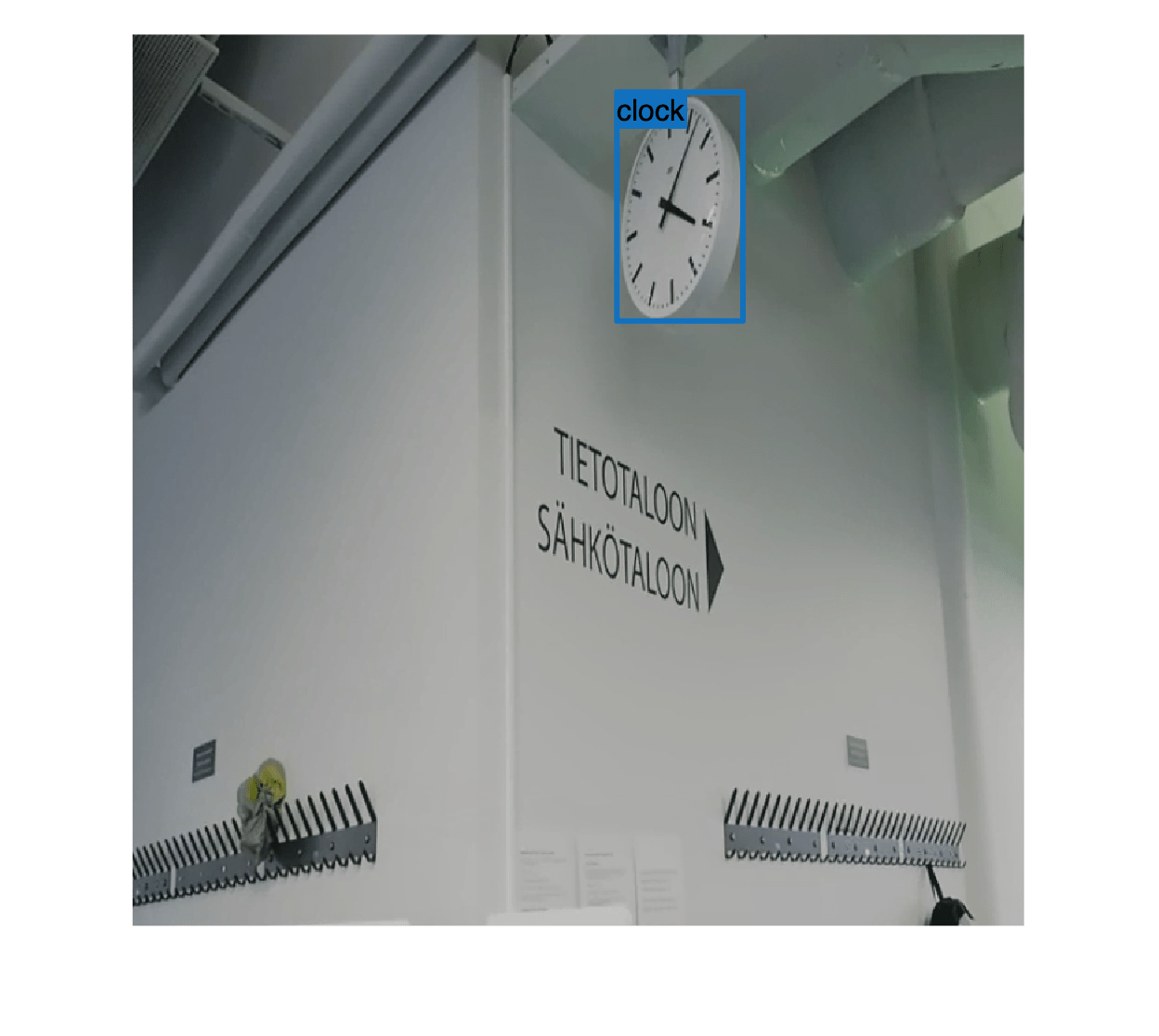### Train YOLOv2 Object Detector

Use `trainingOptions` to specify network training options.

```opts = trainingOptions("rmsprop",... InitialLearnRate=0.001,... MiniBatchSize=8,... MaxEpochs=10,... LearnRateSchedule="piecewise",... LearnRateDropPeriod=5,... VerboseFrequency=30, ... L2Regularization=0.001,... ValidationData=dsVal, ... ValidationFrequency=50, ... OutputNetwork="best-validation-loss");```

These training options were selected using Experiment Manager. For more information on using Experiment Manager for hyperparameter tuning, see Train Object Detectors in Experiment Manager.

Use `trainYOLOv2ObjectDetector` function to train YOLO v2 object detector if `doTraining` is true.

```doTraining = false; if doTraining [detector, info] = trainYOLOv2ObjectDetector(augmentedTrainingData,lgraph, opts); end```

This example was verified on an NVIDIA™ GeForce RTX 3090 Ti GPU with 24 GB of memory. If your GPU has less memory, you may run out of memory. If this happens, lower the `MiniBatchSize` using the `trainingOptions` function. Training this network took approximately 45 minutes using this GPU. Training time varies depending on the hardware you use.

### Evaluate Object Detector

Evaluate the trained object detector on test images to measure the performance. Computer Vision Toolbox™ provides an object detector evaluation function (`evaluateObjectDetection`) to measure common metrics such as average precision and log-average miss rate. For this example, use the average precision metric to evaluate performance. The average precision provides a single number that incorporates the ability of the detector to make correct classifications (precision) and the ability of the detector to find all relevant objects (recall).

Run the detector on the test dataset. Set the detection threshold to a low value to detect as many objects as possible. This helps you evaluate the detector precision across the full range of recall values.

```detectionThreshold = 0.01; results = detect(detector,dsTest, MiniBatchSize=8, Threshold=detectionThreshold);```

Calculate object detection metrics on the test set results with `evaluateObjectDetection`, which evaluates the detector at one or more intersection-over-union (IoU) thresholds. The IoU threshold defines the amout of overlap required between a predicted bounding box and a ground truth bounding box for the predicted bounding box to count as a true positive.

```iouThresholds = [0.5 0.75 0.9]; metrics = evaluateObjectDetection(results, dsTest, iouThresholds);```

List the overall class metrics and inspect the mean average precision (mAP) to see how well the detector is performing. Then, visualize the average precision values across all IoU thresholds.

`metrics.ClassMetrics `
```ans=7×5 table NumObjects mAP AP Precision Recall __________ _______ ____________ ________________ ________________ chair 168 0.60842 {3×1 double} {3×13754 double} {3×13754 double} clock 23 0.551 {3×1 double} {3×2744 double} {3×2744 double} exit 52 0.55121 {3×1 double} {3×3149 double} {3×3149 double} fireextinguisher 165 0.5417 {3×1 double} {3×4787 double} {3×4787 double} printer 7 0.14627 {3×1 double} {3×4588 double} {3×4588 double} screen 4 0.08631 {3×1 double} {3×10175 double} {3×10175 double} trashbin 17 0.26921 {3×1 double} {3×7881 double} {3×7881 double} ```
```figure classAP = metrics.ClassMetrics{:,"AP"}'; classAP = [classAP{:}]; bar(classAP') xticklabels(metrics.ClassNames) ylabel("AP") legend(string(iouThresholds) + " IoU")```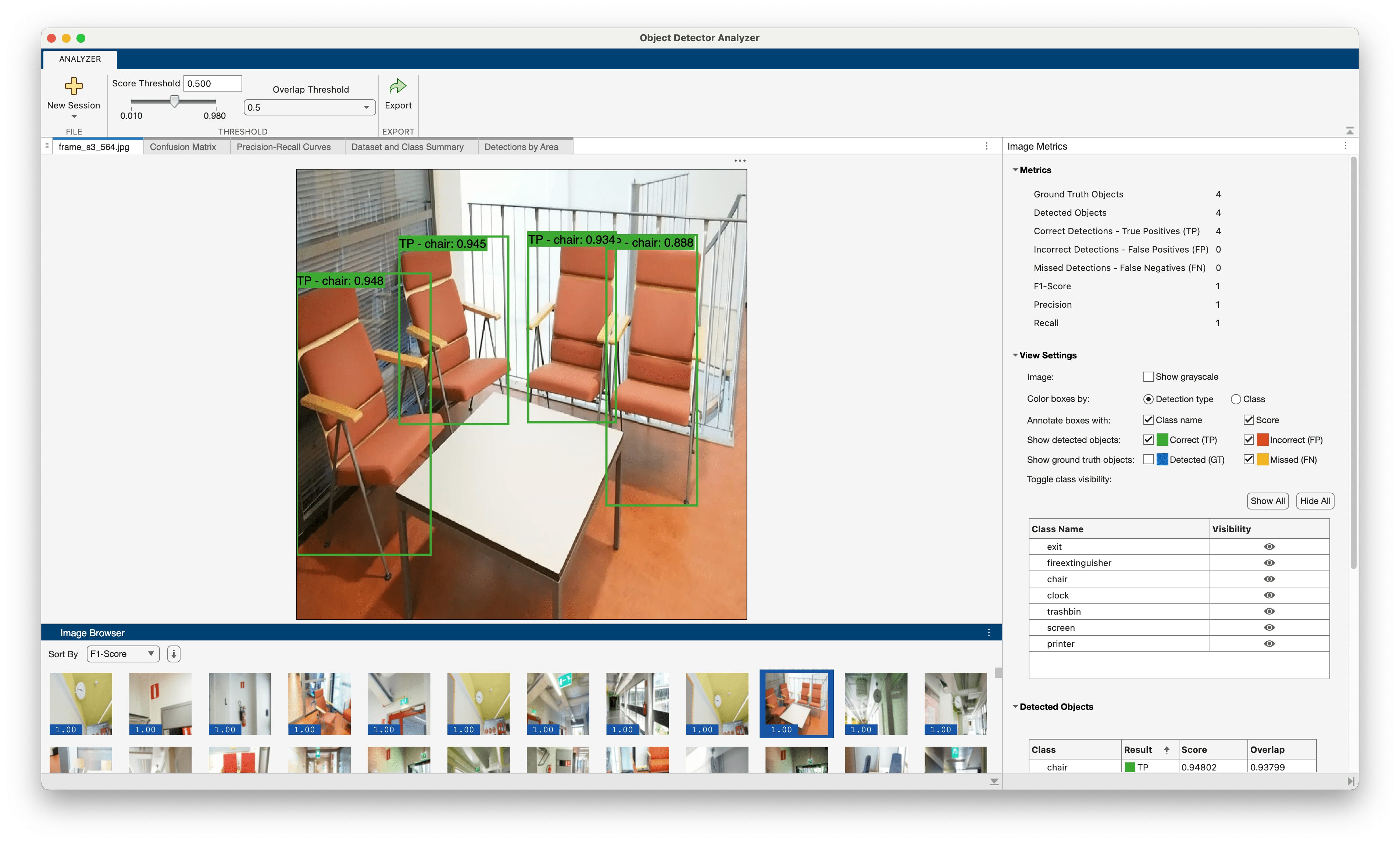The detector did poorly on 3 classes (printer, screen, and trashbin) that had fewer samples compared to the other classes. The performance also degraded at higher IoU thresholds. Based on these results, the next step towards improving performance is to address the class imbalance problem identified earlier in this example by adding more images that containing the underrepresented classes or by replicating images with these classes and using data augmentation. These next steps require additional experiments and are beyond the scope of this example.

Next, investigate the impact object size has on detector performance with `metricsByArea`, which computes detector metrics for specific object size ranges. You can define the size range based on a predefined set of size ranges for your application or you can use the estimated anchor boxes. The anchor box estimation process automatically clusters the object sizes and provides a data-centric set of size ranges.

Extract the anchor boxes from the detector, calculate their areas, and sort the areas.

```areas = prod(detector.AnchorBoxes,2); areas = sort(areas);```

Form area range limits using the calculated areas. The upper limit for the last range is set to 3 times the size of the largest area, which is sufficient for the objects in this dataset.

```lowerLimit = [0;areas]; upperLimit = [areas; 3*areas(end)]; areaRanges = [lowerLimit upperLimit]```

Run `metricsByArea` for the "chair" class.

```classes = string(detector.ClassNames); areaMetrics = metricsByArea(metrics,areaRanges,ClassName=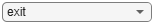classes(3))```
```areaMetrics=6×6 table AreaRange NumObjects mAP AP Precision Recall ________________________ __________ _______ ____________ _______________ _______________ 0 2774 0 0 {3×1 double} {3×152 double} {3×152 double} 2774 9177 19 0.51195 {3×1 double} {3×578 double} {3×578 double} 9177 15916 11 0.21218 {3×1 double} {3×2404 double} {3×2404 double} 15916 47799 43 0.72803 {3×1 double} {3×6028 double} {3×6028 double} 47799 1.2472e+05 74 0.62831 {3×1 double} {3×4174 double} {3×4174 double} 1.2472e+05 3.7415e+05 21 0.60897 {3×1 double} {3×423 double} {3×423 double} ```

Although the detector performed well on the "chair" class overall, there is a size range where detector has a lower average precision compared to the other size ranges. The `NumObjects` column shows how many objects in the test dataset fall within the area range. Here, the range where the detector does not perform well has only 11 samples. Improving the performance further in this size range may require adding more samples of that size or using data augmentation to create more samples across the set of size ranges.

You can repeat this procedure for the other classes to gain deeper insight into how to further improve detector performance.

Finally, plot the precision/recall (PR) curve and the detection confidence scores side-by-side. The precision/recall curve highlights how precise a detector is at varying levels of recall for each class. By plotting the detector scores next to the PR curve, you can choose a detection threshold to achieve a desired precision and recall for your application.

Choose a class, extract the precision and recall metrics for that class, and then plot the precision and recall curves.

```class =classes(3); % Extract precision and recall values. precision = metrics.ClassMetrics{class,"Precision"}; recall = metrics.ClassMetrics{class,"Recall"}; % Plot precision/recall curves. figure tiledlayout(1,2) nexttile plot(recall{:}',precision{:}') ylim([0 1]) xlim([0 1]) grid on xlabel("Recall") ylabel("Precision") title(class + " Precision/Recall ") legend(string(iouThresholds) + " IoU",Location="south")```

Next, extract all the labels and scores from the test set detection results and sort the scores corresponding to the selected class. This reorders the scores to match the order used while computing precision/recall values. This enables visualizing precision/recall and scores side-by-side.

```allLabels = vertcat(results{:,3}{:}); allScores = vertcat(results{:,2}{:}); classScores = allScores(allLabels == class); classScores = [1;sort(classScores,'descend')];```

Visualize the scores next to the precision/recall curves.

```nexttile plot(recall{1,:}',classScores) ylim([0 1]) xlim([0 1]) ylabel("Score") xlabel("Recall") grid on title(class + " Detection Scores")```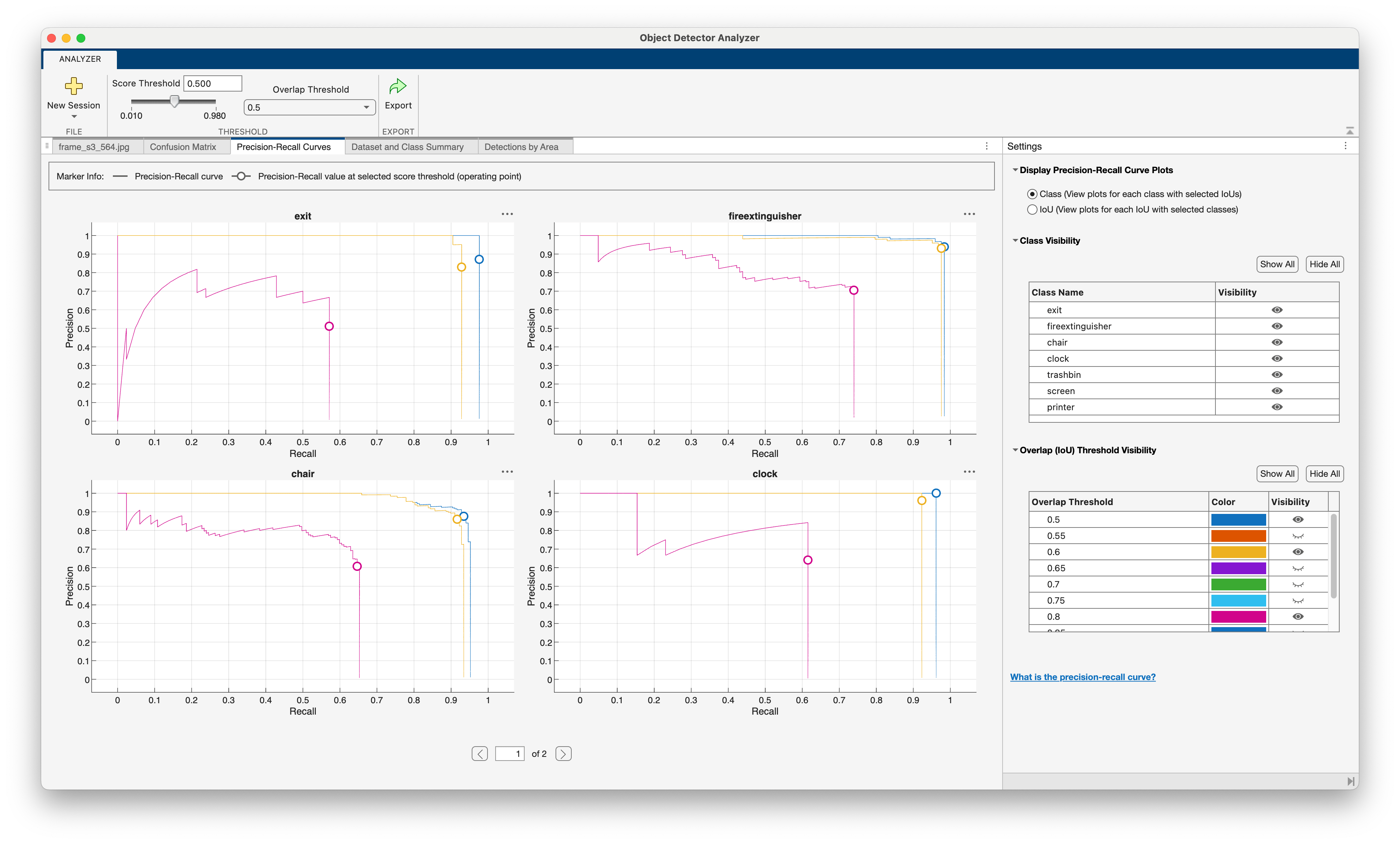As the figure shows, the detection threshold lets you trade-off precision for recall. Choose a threshold that gives you the precision/recall characteristics best suited for your application. For example, at an IoU threshold of 0.5, you can achieve a precision of 0.9 at a recall level of 0.9 for the chair class by setting the detection threshold to 0.4. You must analyze precision/recall curves for all the classes before choosing a final detection threshold because the precision/recall characteristics may be different for each class.

### Deployment

Once the detector is trained and evaluated, you can generate code and deploy the `yolov2ObjectDetector` using GPU Coder™. See Code Generation for Object Detection by Using YOLO v2 (GPU Coder) example for more details.

### Summary

This example shows how to train and evaluate a multiclass object detector. When adapting this example to your own data, carefully assess the object class and size distribution in your dataset. Your data may require using a different hyperparameters or a different object detector such as YOLO v4 or YOLO X for optimal results.

### Supporting Functions

```function B = augmentData(A) % Apply random horizontal flipping, and random X/Y scaling. Boxes that get % scaled outside the bounds are clipped if the overlap is above 0.25. Also, % jitter image color. B = cell(size(A)); I = A{1}; sz = size(I); if numel(sz)==3 && sz(3) == 3 I = jitterColorHSV(I,... Contrast=0.2,... Hue=0,... Saturation=0.1,... Brightness=0.2); end % Randomly flip and scale image. tform = randomAffine2d(XReflection=true, Scale=[1 1.1]); rout = affineOutputView(sz, tform, BoundsStyle="CenterOutput"); B{1} = imwarp(I, tform, OutputView=rout); % Sanitize boxes, if needed. This helper function is attached as a % supporting file. Open the example in MATLAB to open this function. A{2} = helperSanitizeBoxes(A{2}); % Apply same transform to boxes. [B{2},indices] = bboxwarp(A{2}, tform, rout, OverlapThreshold=0.25); B{3} = A{3}(indices); % Return original data only when all boxes are removed by warping. if isempty(indices) B = A; end end```
```function data = resizeImageAndLabel(data,targetSize) % Resize the images and scale the corresponding bounding boxes. scale = (targetSize(1:2))./size(data{1},[1 2]); data{1} = imresize(data{1},targetSize(1:2)); data{2} = bboxresize(data{2},scale); data{2} = floor(data{2}); imageSize = targetSize(1:2); boxes = data{2}; % Set boxes with negative values to have value 1. boxes(boxes<=0) = 1; % Validate if bounding box in within image boundary. boxes(:,3) = min(boxes(:,3),imageSize(2) - boxes(:,1)-1); boxes(:,4) = min(boxes(:,4),imageSize(1) - boxes(:,2)-1); data{2} = boxes; end```

### References

 Adhikari, Bishwo; Peltomaki, Jukka; Huttunen, Heikki. (2019). Indoor Object Detection Dataset [Data set]. 7th European Workshop on Visual Information Processing 2018 (EUVIP), Tampere, Finland.

 Lin, Tsung-Yi, Michael Maire, Serge Belongie, Lubomir Bourdev, Ross Girshick, James Hays, Pietro Perona, Deva Ramanan, C. Lawrence Zitnick, and Piotr Dollár. “Microsoft COCO: Common Objects in Context,” May 1, 2014. https://arxiv.org/abs/1405.0312v3.